Next: Velocity Potentials and Stream Up: Two-Dimensional Incompressible Inviscid Flow Previous: Introduction

# Two-Dimensional Flow

Fluid motion is said to be two-dimensional when the velocity at every point is parallel to a fixed plane, and is the same everywhere on a given normal to that plane. Thus, in Cartesian coordinates, if the fixed plane is the-plane then we can express a general two-dimensional flow pattern in the form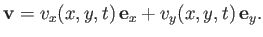(5.1)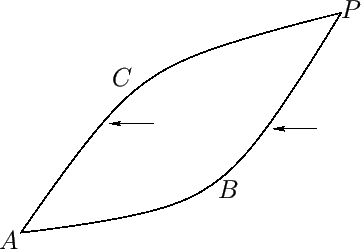Letbe a fixed point in the-plane, and let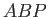and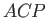be two curves, also in the-plane, that jointo an arbitrary point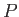. (See Figure 5.1.) Suppose that fluid is neither created nor destroyed in the region,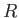(say), bounded by these curves. Because the fluid is incompressible, which essentially means that its density is uniform and constant, fluid continuity requires that the rate at which the fluid flows into the region, from right to left (in Figure 5.1) across the curve, is equal to the rate at which it flows out the of the region, from right to left across the curve. The rate of fluid flow across a surface is generally termed the flux. Thus, the flux (per unit length parallel to the-axis) from right to left acrossis equal to the flux from right to left across. Becauseis arbitrary, it follows that the flux from right to left across any curve joining pointsandis equal to the flux from right to left across. In fact, once the base pointhas been chosen, this flux only depends on the position of point, and the time. In other words, if we denote the flux bythen it is solely a function of the location ofand the time. Thus, if pointlies at the origin, and pointhas Cartesian coordinates (,), then we can write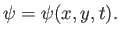(5.2)

The functionis known as the stream function. Moreover, the existence of a stream function is a direct consequence of the assumed incompressible nature of the flow.

Consider two points,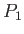and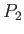, in addition to the fixed point. (See Figure 5.2.) Let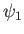and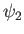be the fluxes from right to left across curves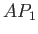and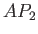. Using similar arguments to those employed previously, the flux acrossis equal to the flux acrossplus the flux across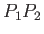. Thus, the flux across, from right to left, is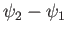. Ifandboth lie on the same streamline then the flux acrossis zero, because the local fluid velocity is directed everywhere parallel to. It follows that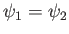. Hence, we conclude that the stream function is constant along a streamline. The equation of a streamline is thus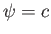, where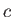is an arbitrary constant.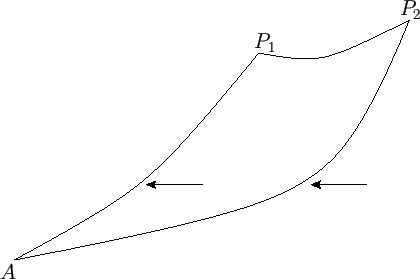Let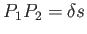be an infinitesimal arc of a curve that is sufficiently short that it can be regarded as a straight-line. The fluid velocity in the vicinity of this arc can be resolved into components parallel and perpendicular to the arc. The component parallel to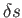contributes nothing to the flux across the arc from right to left. The component perpendicular tocontributes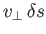to the flux. However, the flux is equal to. Hence,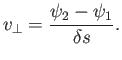(5.3)

In the limit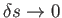, the perpendicular velocity from right to left across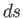becomes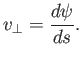(5.4)

Thus, in Cartesian coordinates, by considering infinitesimal arcs parallel to the- and-axes, we deduce that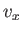(5.5)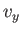(5.6)

These expressions can be combined to give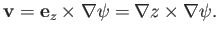(5.7)

Note that when the fluid velocity is written in this form then it immediately becomes clear that the incompressibility constraint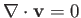is automatically satisfied [because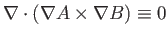--see Equations (A.175) and (A.176)]. It is also clear that the stream function is undefined to an arbitrary additive constant.

The vorticity in two-dimensional flow takes the form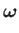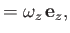(5.8)

where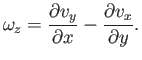(5.9)

Thus, it follows from Equations (5.5) and (5.6) that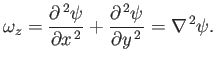(5.10)

Hence, irrotational two-dimensional flow is characterized by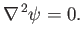(5.11)

When expressed in terms of cylindrical coordinates (see Section C.3), Equation (5.7) yields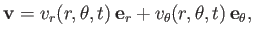(5.12)

where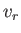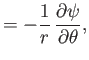(5.13)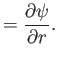(5.14)

Moreover, the vorticity is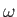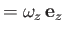, where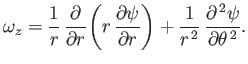(5.15)Next: Velocity Potentials and Stream Up: Two-Dimensional Incompressible Inviscid Flow Previous: Introduction
Richard Fitzpatrick 2016-03-31